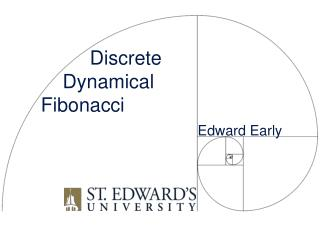DownloadDownload PresentationDiscrete Dynamical Fibonacci

# Discrete Dynamical Fibonacci

Télécharger la présentation## Discrete Dynamical Fibonacci

- - - - - - - - - - - - - - - - - - - - - - - - - - - E N D - - - - - - - - - - - - - - - - - - - - - - - - - - -
##### Presentation Transcript

1. Discrete Dynamical Fibonacci Edward Early

2. Fibonacci Numbers • F0 = 0, F1= 1, Fn=Fn-1+Fn-2for n>1 • 0, 1, 1, 2, 3, 5, 8, 13, 21, 34, 55, 89, 144, …

3. Differential Equations • inspired by undetermined coefficients • Fn = Fn-1+Fn-2characteristic polynomialx2-x-1 • formula • initial conditions F0= 0, F1 = 1giveC1andC2 • BIG DRAWBACK: students were already taking undetermined coefficients on faith (and this application not in book)

4. Linear Algebra • discrete dynamical systems whereλ1andλ2are the (distinct) eigenvalues of the2×2matrixAwith eigenvectorsv1andv2, respectively, and x0=c1v1+c2v2 (Section 5.6 of Lay’s book)

5. Linear Algebra Meets Fibonacci • Let and • Thus Anx0has top entry Fn

6. Linear Algebra Meets Fibonacci • Let and • det(A-λI) = λ2-λ-1 • eigenvalues and • eigenvectors and

7. Linear Algebra Meets Fibonacci • Let and • top entry

8. Caveat • If then… • ugly enough to scare off most students!